Courses

# SpA Test (14-10-2018)

## 60 Questions MCQ Test | SpA Test (14-10-2018)

Description
This mock test of SpA Test (14-10-2018) for Chemistry helps you for every Chemistry entrance exam. This contains 60 Multiple Choice Questions for Chemistry SpA Test (14-10-2018) (mcq) to study with solutions a complete question bank. The solved questions answers in this SpA Test (14-10-2018) quiz give you a good mix of easy questions and tough questions. Chemistry students definitely take this SpA Test (14-10-2018) exercise for a better result in the exam. You can find other SpA Test (14-10-2018) extra questions, long questions & short questions for Chemistry on EduRev as well by searching above.
QUESTION: 1

### The rate of a chemical reaction doubles for every 10°C rise of temperature. If the temperature is raised by 50°C, the rate of the reaction increases by about:

Solution: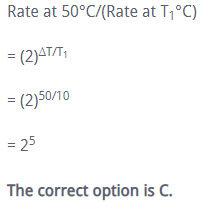QUESTION: 2

### The rate of the reaction A → products, at the initial concentration of 3.24 × 10–2 M is nine times its rate at another initial concentration of 1.2 × 10–3 M. The order of the reaction is:

Solution: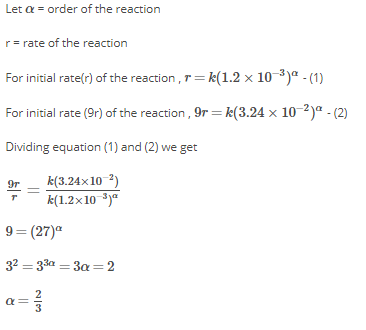QUESTION: 3

### For the reaction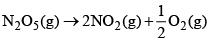the value of rate of disappearance of N2O5 is given as 6.25 × 10–3mol L–1S–1. The rate of formation of NO2 and O2 is given respectively as:

Solution:

-d[N2O5]/dt = +2d[NO2]/dt = 1/2d[O2]/dt

rate of formation of NO2 = (rate of disappearence of N2O5)/2

rate of formation of NO2 = 6.25x10-3/2 = 3.125x103

rate of formation of O2=rate of disappearence of N2O5)2

rate of formation of O2

= 6.25*10-3x2

=1.25x10-2

QUESTION: 4

In the synthesis of ammonia by Haber process, if 60 moles of ammonia is obtained in one hour, then the rate of disappearance of Nitrogen is:

Solution:
QUESTION: 5

For a first order reaction A → P, the temperature (T) dependent rate constant (k) was found to follow the equation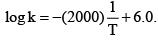The pre-exponential factor A and the activation energy Ea, respectively, are:

Solution:

The logarithmic form of Arrhenius equation is

logk = log A−Ea 2.303 RT

Given, log k = 6 − 2000 T

Comparing the above two equations :

logA=6⇒A=106

and Ea 2.303 R=2000

⇒Ea=2000×2.303×8.314J

=38.3 kJmol−1

Thus the activation energy is 38.3 kJmol−1

QUESTION: 6

Deoxy-Hemocyanin is:

Solution:
QUESTION: 7

The biological functions of the cytochrome P450 and myoglobin are, respectively:

Solution:
QUESTION: 8

Which statement is the correct description of hemerythrin:

Solution:
QUESTION: 9

Which of the following is a heme iron protein:

Solution:
QUESTION: 10

The active site of enzyme nitrogenase contains:

Solution:

The most abundant and widely studied nitrogenase group is the Mo-dependent enzyme, which has an active site metallo-cofactor called FeMo-cofactor

QUESTION: 11

A quantity of 0.50 mole of an ideal gas at 20°C expands isothermally against a constant pressure of 2.0 atm from 1.0 L to 5.0 L. Entropy change of the system is:

Solution:
QUESTION: 12

Given the molecular formula of the hexa coordinated complexes (A) CoCl3.6NH3 (B) CoCl3.5NH3 (C) CoCl3.4NH3. If the number of coordinated NH3 molecules in A, B and C respectively are 6, 5 and 4 the primary valency in (A), (B) and (C) are:

Solution:

The primary valencies in (i) CoCl3.6NH3, (ii) CoCl3.5NH3, (iii) CoCl3.4NH3. If the number of co-ordinated NH3 molecules in i, ii and iii respectively are 6, 5, 4,(i), (ii) and (iii) are 3, 2 and 1 respectively.

(i) CoCl3.6NH3 in this complex Co is co-ordinated with six NH3 molecules, which means the primary valency of Co is 3. complex is [Co(NH3)6]Cl3

(ii) CoCl3.5NH3 in this complex Co is co-ordinated with five NH3 molecules, which means the primary valency of Co is 2.complex is [Co(NH3)5]Cl2

(iii) CoCl3.4NH3 in this complex Co is co-ordinated with four NH3 molecules, which means the primary valency of Co is 1.complex is [Co(NH3)4]Cl

QUESTION: 13

Ketones react with Mg-Hg over water gives:

Solution:

This reaction involves the reductive homo-coupling of a carbonyl compound to produce a symmetrically substituted 1,2-diol. The first step is single electron transfer of the carbonyl bond, which generates radical ion intermediates that couple via carbon-carbon bond formation to give a 1,2-diol. The example depicted above shows the preparation of pinacol itself.

Pinacol and other highly substituted 1,2-diols tend to undergo dehydration with rearrangement under acid-catalysis.

QUESTION: 14

Which one of the following statement for order of reaction is not correct:

Solution:

Out of all four statements option D is not correct since an order of reaction can be 1, 2, 3 ... and even fractional also.

Also, an order can be determined experimentally and if is not affected by the stoichiometric coefficient of the reactants.

QUESTION: 15

t1/4 can be taken as the time taken for the concentration of a reactant to drop to 3/4 of its initial value. If the rate constant for a first order reaction is K, the t1/4 can be written as:

Solution: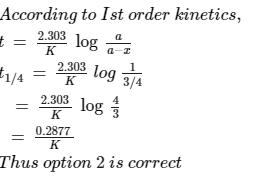QUESTION: 16

The compound that undergoes decarboxylation most readily under mild condition is:

Solution:

Beta keto acids readily undergo thermal decarboxylation. The compound that undergoes decarboxylation most readily under mild condition is 2-oxocyclohexanecarboxylic acid (option B) as it is a beta keto acid. The product obtained is cyclohexanone.

QUESTION: 17

Consider the acidity of the carboxylic acids:

(I) PhCOOH
(II) o-NO2C6H4COOH
(III) p-NO2C6H4COOH
(IV) m-NO2C6H4COOH

Which of the following order is correct:

Solution:
QUESTION: 18

Starting with one mole of a compound A it is found that the reaction is 3/4th complete in one hour. If the reaction is first order, the rate constant is:

Solution:
QUESTION: 19

Find the organic acid produced from the below reaction: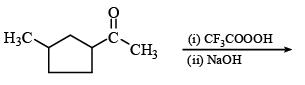Solution:
QUESTION: 20

The diketone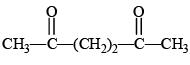on intramolecular aldol condensation gives the final product.

Solution:
QUESTION: 21

Match the following: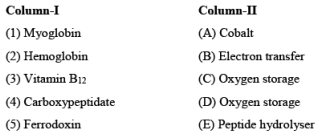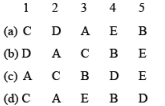Solution:
QUESTION: 22

Which of the following does not have optical isomer

Solution:

The complex [Co(NH3​)3​Cl3​] does not have optical isomers due to presence of an element of symmetry. However, it exists in two geometrical isomers which are cis and trans or fac and mer isomers.

QUESTION: 23

Which one is not correct:

Solution:
QUESTION: 24

Which of the following complex ions is expected to absorb visible light:

Solution:

(a) In [Sc(H2​O)(NH3​)3​]3+ Sc is present in Sc3+=[Ar]3d0,4s0

Since in this complex excitation of the electron is not possible, it will not absorb visible light.

(b) [Ti(en)2​(NH3​)2​]4+, Ti is present as Ti4+

Ti4+=[Ar]3d0,4s0

Hence it will not absorb visible light

(c) In [Cr(NH3​)6​]3+ Cr is present as Cr3+

Cr =[Ar]3d3,4s

Since this complex has three unpaired electrons excitation of electrons is possible and thus, it is expected that this complex will absorb visible light.

(d) In [Zn(NH3​)6​]2+ Zn is present as Zn2+

Zn2+=[Ar]3d10,4s0

Hence , this complex will not absorb visible light.

The correct option is C.

QUESTION: 25

The reaction [Fe(CNS)6]3– → [FeF6]3– takes place with:

Solution:

[Fe(CNS)6​]3−→[FeF6​]3−

CNS is  a strong ligand while  F is a weak ligand . Outer orbital configuration from inner orbital is achieved. hence it causes more spin of electrons. Hence it results in increased magnetic moment.

QUESTION: 26

The crystal field stabilization energy (CFSE) is the highest for:

Solution:

Higher the oxidation state of the metal, greater the crystal field splitting energy . In options (a), (b) and (d), Co is present in +2 oxidation state and in (c ) it is present in +3 oxidation state and hence has a higher value of CFSE.

QUESTION: 27

CH3COOCH3 excess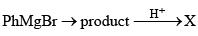The product X is

Solution:
QUESTION: 28

An optically active compound having molecular formula C8H16 on ozonolysis gives acetone as one of the products. The structure of the compound is:

Solution:
QUESTION: 29

The major organic product formed from the following reaction: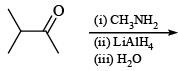Solution:
QUESTION: 30

For the redox reaction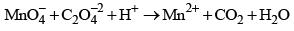The correct coefficients of the reaction for the balanced reaction are:Solution:
*Multiple options can be correct
QUESTION: 31

For a first order reaction:

Solution:

For a first order reaction, A→ Products, we have

In[A]/ ​[A]0​​=−kt or[A]t​=[A]0​e−kt

In terms of degree of dissociation,

[A]t​ = [A]0​(1−α)

Hence, [A]0​(1−α) = [A]0​e−kt
or α = 1−e−kt

The Arrehnius equation is k = Ae−Ea​/RT

The dimension of pre-exponential factor A in the above expression is the same as that of rate constant k. For a first order reaction, the dimension of k is t−1

*Multiple options can be correct
QUESTION: 32

The following statement(s) is(are) correct:

Solution:
*Multiple options can be correct
QUESTION: 33

For the first order reaction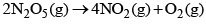Solution: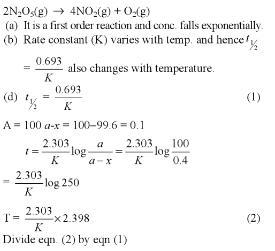*Multiple options can be correct
QUESTION: 34

The rate of law for the reaction.

RCl + NaOH → ROH + NaCl

is given by rate = k[RCl]. The rate of this reaction

Solution:
*Multiple options can be correct
QUESTION: 35

For the reaction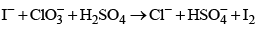The correct statement(s) in the balanced equation is/are:

Solution:

I+ClO3​+H2​SO4​⟶Cl+HSO4​+I2

→ I gets oxidised.

→ SO4​ neither gets oxidised nor reduced.

→ H2​O is produced.

Balance equation:

6I+ClO3​+6H2​SO4​⟶Cl+6HSO4​+3I2​+3H2​O

∴ Stoichiometric coefficient of HSO4−​ is 6.

*Multiple options can be correct
QUESTION: 36

Reduction of the metal centre in aqueous permangnate ion involves:

Solution:

The reactions of permanganate ion in different media are given below:
In acidic medium:
MnO4​+8H++5e→Mn2++2H2​O
In neutral medium:
MnO4​+2H2​O+3e→MnO2​+4OH
In alkaline medium:
MnO4​+e→MnO42−
Hence, number of electrons transfer in acidic, neutral and alkaline medium are 5,3 and 1 respectively.

*Multiple options can be correct
QUESTION: 37

Radiocarbon is produced in the atmosphere as a result of

Solution:

Radiocarbon is produced in the atmosphere as result of collision between fast neutrons and nitrogen nuclei present in the atmosphere.
Nuclear reaction is given as :
7​N14 + 0​n1 → 6​C14 + 1​H1

*Multiple options can be correct
QUESTION: 38

In biological system, the metal ion involved in the dioxygen transport besides Fe is:

Solution:
*Multiple options can be correct
QUESTION: 39

Which one of the following is wrongly matched:

Solution:
*Multiple options can be correct
QUESTION: 40

Red precipitate is obtained when ethanol solution of dimethylglyoxime is added to ammonicalNi(II). Which of the following statement is/are true: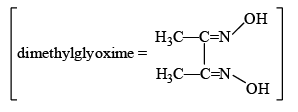Solution:
*Answer can only contain numeric values
QUESTION: 41

If the activation energy for the forward reaction is 150 kJ mol–1 and that of the reverse reaction is 260 kJ mol–1, what is the enthalpy change (kJ mol–1) for the reaction ?

Solution:

For a reversible reaction ,
ΔH= Ea (forward) − Ea (backward)
ΔH=150−260=−110kJmol−1

*Answer can only contain numeric values
QUESTION: 42

The rate of a reaction is doubled for every 10°C rise in temperature. The increase in reaction rate as a result of temperature rise from 10°C to 100°C is?

Solution:

The rate of the reaction is doubled for every 10oC rise in Temperature.

So the increase in reaction rate as a result of rise in temperature from 10oC to 100oC is 29 = 512 times

*Answer can only contain numeric values
QUESTION: 43

Cyclopropene rearranges to form propene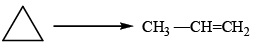This follows first order kinetics. The rate constant is 2.714 × 10–3 sec–1. The initial concentration of cyclopropane is 0.29 M. What will be the concentration (M) of cyclcopropene after 100 sec ? (answer upto two decimal places)

Solution:

k=(2.303/t) log(a/(a−x))

(a-x) is the concentration left after 100 sec .

2.7×10−3=(2.303/100)log(0.29/(a−x))

⇒(a−x)=0.22M

*Answer can only contain numeric values
QUESTION: 44

A gaseous hypothetical chemical equation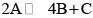is carried out in a closed vessel. The concentration of B is found to increase by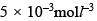in 10 second. The rate of appearance of B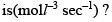(in the multiple 10-4)

Solution:
*Answer can only contain numeric values
QUESTION: 45

1 mole of an ideal gas for which CV = 3/2 R is heated reversibly at a constant pressure of 1 atm from 25°C to 100°C. The ΔH(cal) is approximately ?

Solution:

As (CP−CV)=R⇒CP=CV+R
⇒CP=(3/2 R) +R =(5/2 R)
Heat given at constant pressure
(ΔH)=nCPΔT=1×(5/2 R)×(373−298)
⇒(ΔH)=1×5/2×1.987×75 = 372.56 cal= 373 cal

*Answer can only contain numeric values
QUESTION: 46

Number of correct statements among following, regarding hemoglobin are ?
(I) Binding with O2 is weaker in compression with myoglobin
(II) Iron is 5-coordinated
(III) Iron is coplanar with the porphyrin ring in the absence of oxygen
(IV) The oxidation state of iron is +2

Solution:
*Answer can only contain numeric values
QUESTION: 47

The possible number of optical isomers in [Co(en)2Cl2]+ are ?

Solution:

It’s helpful to get a model kit in mind to be able to visualize the octahedral coordination (that way you can rotate these molecules in your hands to see how they fit together)- here’s what the isomers look like: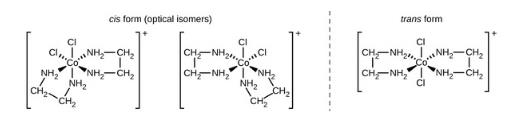Notice that the cis form of the molecule has optical isomers (also known as enantiomers). This is a great example of chirality in inorganic chemistry! If you overlaid the two cis isomers on top of each other, you would find that they’re non-superimposable (they aren’t the same no matter how you rotate them). Meanwhile, the trans form of the molecule does not have any enantiomers.

*Answer can only contain numeric values
QUESTION: 48

The magnetic moment of a transition metal ion is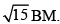Therefore the number of unpaired electrons present in it is ?

Solution:

Magnetic moment =√n(n+2)
where, n = no. of unpaired electrons.
given, μ=√15
∴ √15= √n(n+2)= 15=n(n+2)
This is possible only when n=3

*Answer can only contain numeric values
QUESTION: 49

The spin only magnetic moment value (in Bohr magneton units) of Cr(CO)6 is ?

Solution:

In Cr(CO)6, the central Cr atom is d2sp3 hybridised. Hence all the electrons in its valence shell is paired. So it has net magnetic moment zero (0 BM).

The electron configuration is [Ar]3d54s1.We have to accomodate the 6 Ligands and the fact that CO is a strong ligand.

This results in d2sp3 hybridization. Therefore, there are no unpaired electrons in Cr(CO)6. Hence n=0

And the spin only magnetic moment is also 0.

*Answer can only contain numeric values
QUESTION: 50

The total number of possible isomers for the complex compound [CuII(NH3)4][PtIICl4] are ?

Solution:

The isomers of the complex compound [CuII(NH3)4][PtIICl4] are :

[Cu(NH3)3Cl][Pt(Cl3)NH3] ,[Cu(NH3)2Cl2][PtCl2(NH3)2], [CuNH3Cl3][PtCl(NH3)3], [CuCl4][Pt(NH3)4]

So, the total no. of isomers are = 4.

*Answer can only contain numeric values
QUESTION: 51

The decomposition of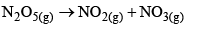Proceeds as a first order reaction with a half-life period of 30 seconds at a certain temperature. If the initial concentration [N2O5] = 0.4 M, what is the rate constant of the reaction(sec-1) ? (answer upto four decimal places)

Solution:
*Answer can only contain numeric values
QUESTION: 52

If a substance with half life 3 days is taken at other place in 12 days. What amount of substance is left now ? (answer upto four decimal places)

Solution:

If it has been 12 days, and the half life is 3 days, then it has been 4 half life cycles. This means you have one half of one half of one half of one half the amount that was there at the start of the 12 day period. 1/2 x 1/2 x 1/2×1/2 = 1/16 = 0.0625 of initial amount

*Answer can only contain numeric values
QUESTION: 53

The rate of reaction between two reactants A and B decreases by a factor of 4 if the concentration of reactant B is doubled. The order of this reaction with respect to reactant B is ?

Solution:

xA + yB ==> Product
Rate= k[A]x.[B]y .........(i)
When conc. of B is doubled,
Rate/4 = k[A]x.[2B]y...........(ii)
Equation (i)/(ii)
4=1/2y
⇒2y = [1/2]²
⇒y= -2 with respect to B

*Answer can only contain numeric values
QUESTION: 54

The rate of disappearance of SO2 in the reaction 2SO2 + O2→ 2SO3 is 1.28 × 10-3 g/sec then the rate of formation (g/sec) of SO3 is ? (in the multiple of 10-3)

Solution:
*Answer can only contain numeric values
QUESTION: 55

The total number of inorganic sulphur (or sulphur) atoms present in the metalloproteins active sites of ruberdoxin, 2-iron ferredoxin and 4-iron ferredoxin, respectively, are ?

Solution:
*Answer can only contain numeric values
QUESTION: 56

Heat obtained due to expansion of 1 mole of H2 gas at 1000 K from 10 L to 100 L under isothermal reversible condition is absorbed by an engine having a sink at 300 K. Useful work (cal) obtained is ?

Solution:
*Answer can only contain numeric values
QUESTION: 57

At constant pressure 200 g of water is heated from 10°C to 20°C. Thus, increase in entropy is: (Given: molar heat capacity of water (Joules) at constant pressure is 75.3 JK–1mol–1) ?

Solution:
*Answer can only contain numeric values
QUESTION: 58

Carnot cycle is said to have 25% efficiency when it operates between T (source) and 300 K (sink), Temperature T is (in K) ?

Solution:
*Answer can only contain numeric values
QUESTION: 59

The value of log10 K for the reaction  A ⇋ B  is ? (Given, Δt298 K = - 54.07 kJ mol-1,
Δt298 K = 10 JK-1mol-1, R = 8.314 JK-1mol-1)  (answer upto two decimal places

Solution:*Answer can only contain numeric values
QUESTION: 60

1 mole of an ideal gas is allowed to expand reversibly and adiabatically from a temperature of 27°C. The work done is 3 kJ. The final temperature (K) of the gas is equal to ? (Given: CV = 20 J mol–1K–1):

Solution:

From first law of thermodynamics,

ΔU=q+W

As we know that, for adiabatic condition,

q=0

∴ΔU=W.....(1)

At constant volume,

ΔU=nCv​ΔT.....(2)

From eqn(1)&(2), we have

W=nCv​ΔT.....(3)

Given:-

Ti​=27℃=(27+273)K=300K

Tf​=T(say)=?

ΔT=Tf​−Ti​=(T−300)

Cv​=20J/K −mol

W=−3kJ=−3×103J[∵Work done by the gas is negative]

Substituting all these values in eqn(3), we have

−3000=1×20×(T−300)

T−300=−150

⇒T=300−150=150K

Hence the final temperature is 150K.

• Test